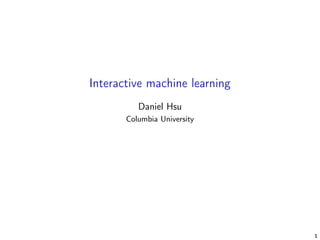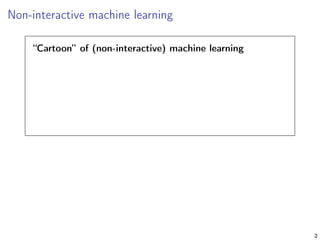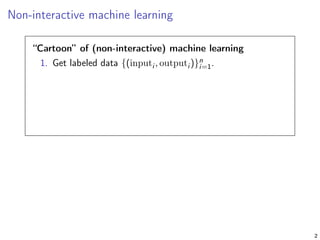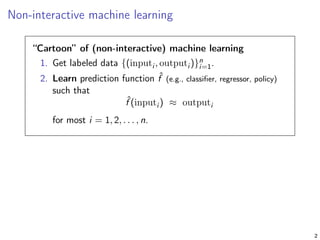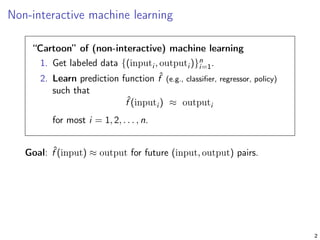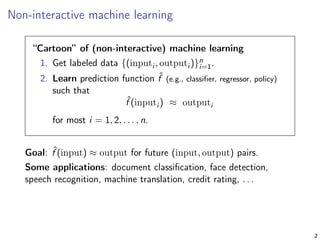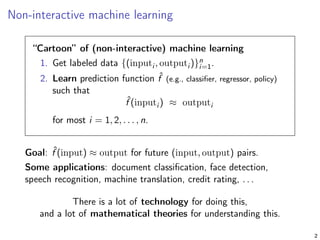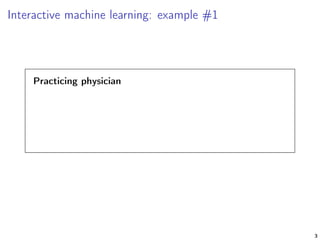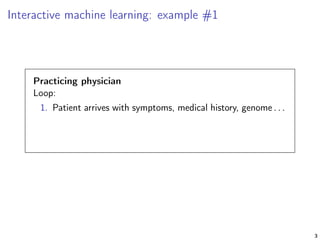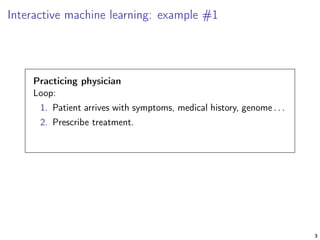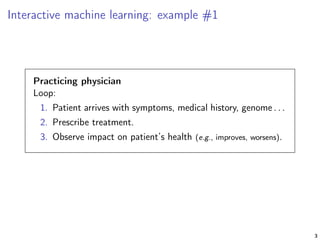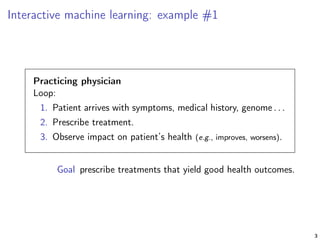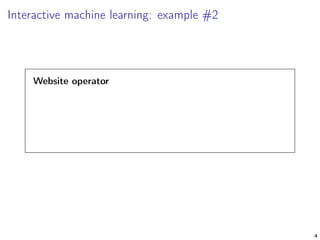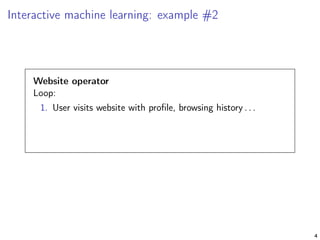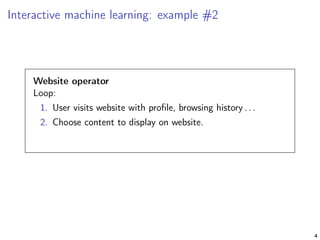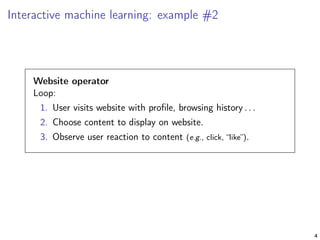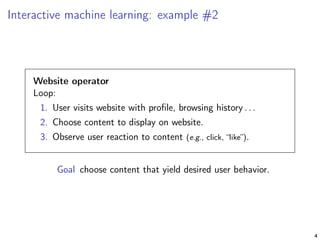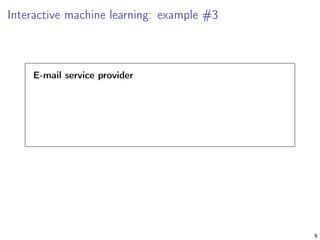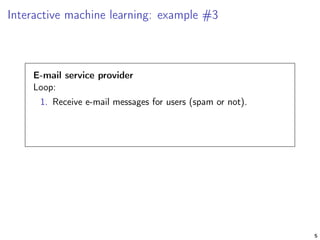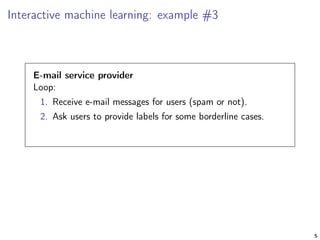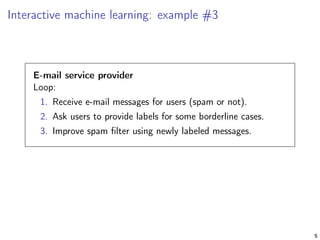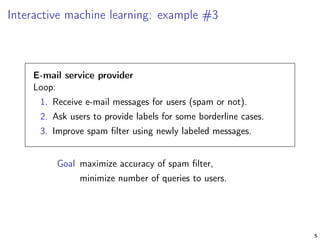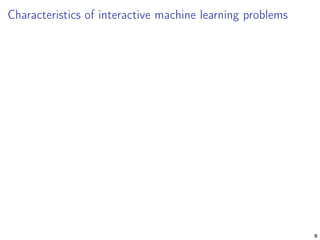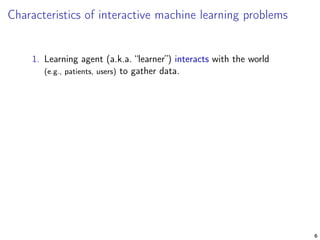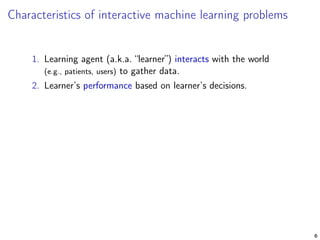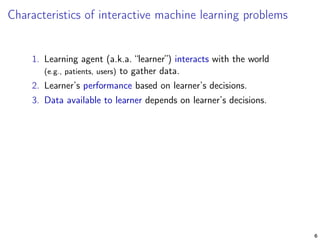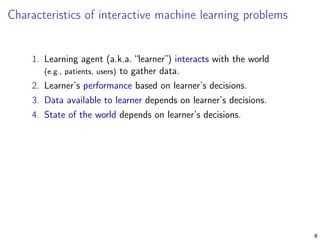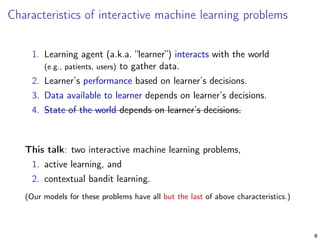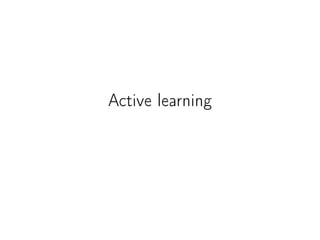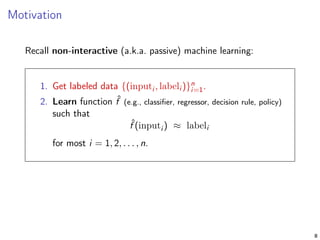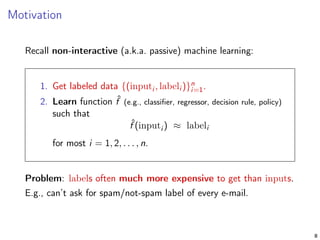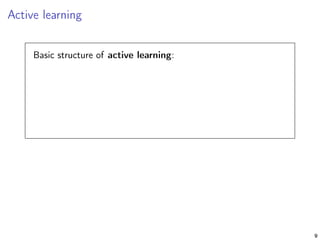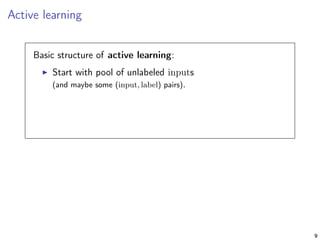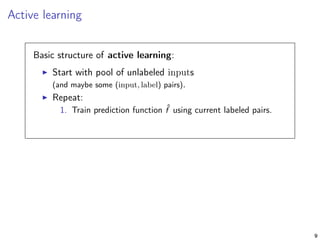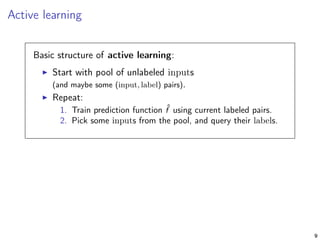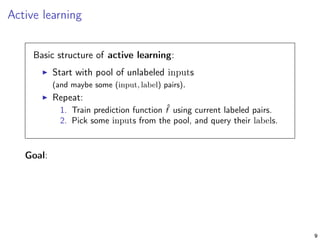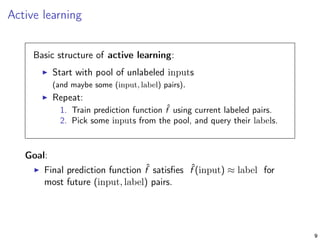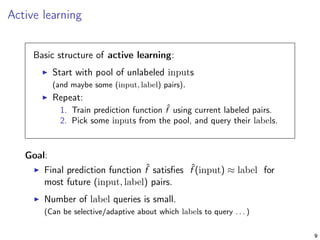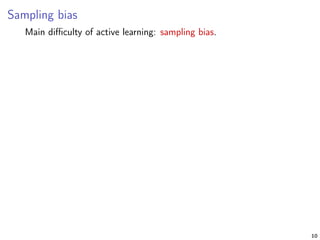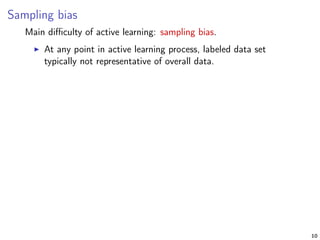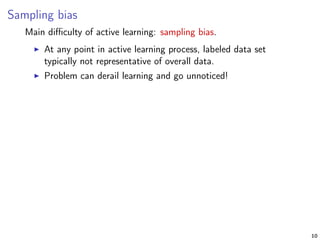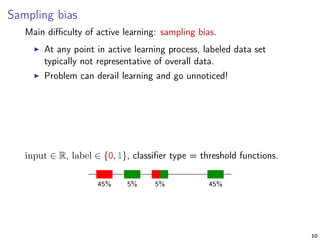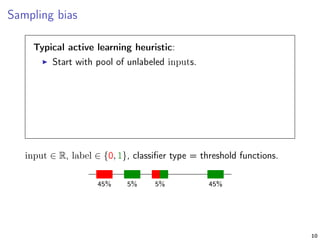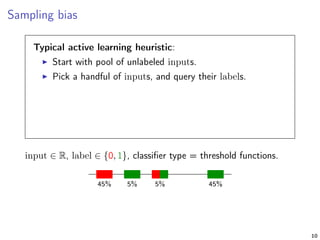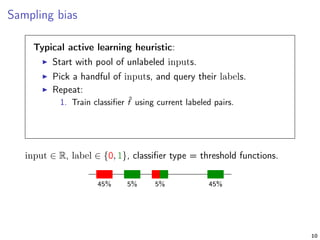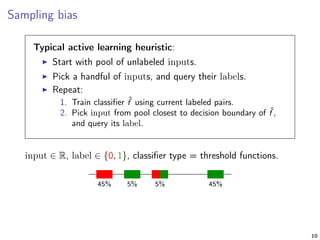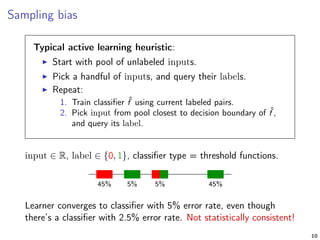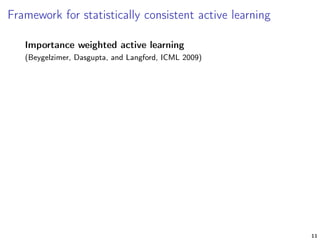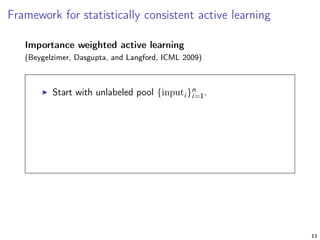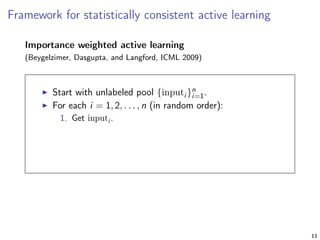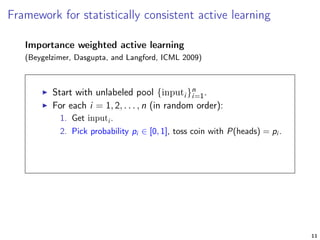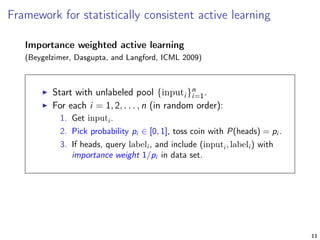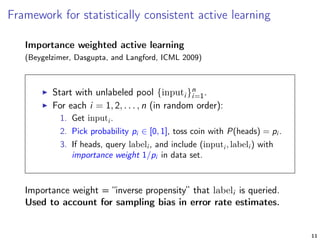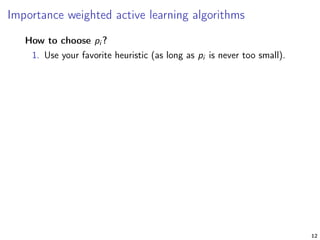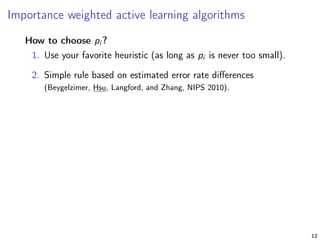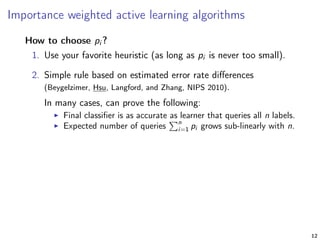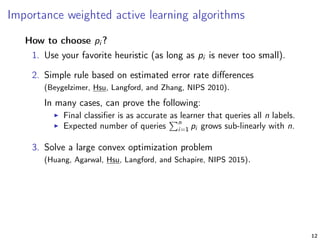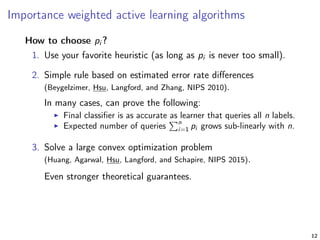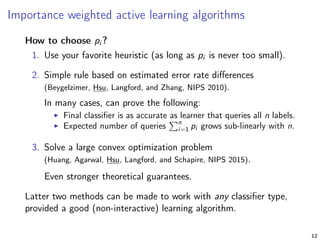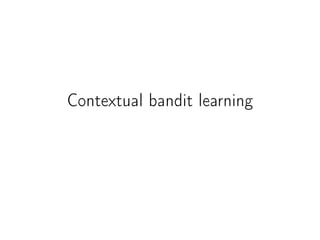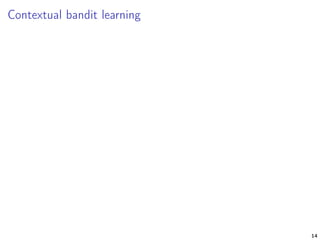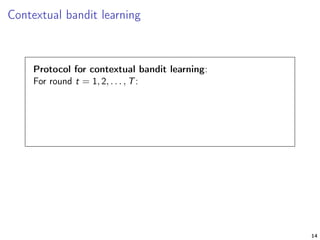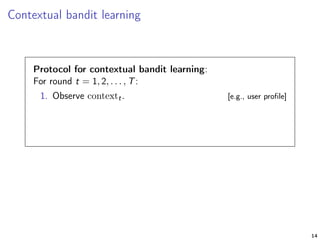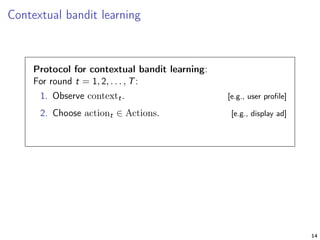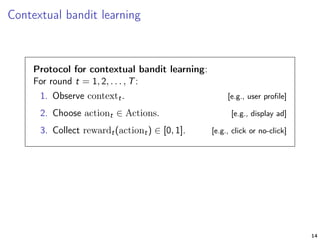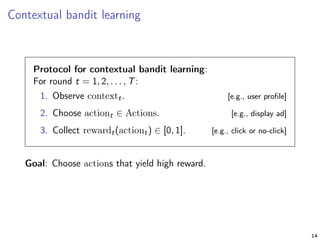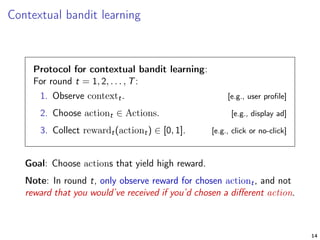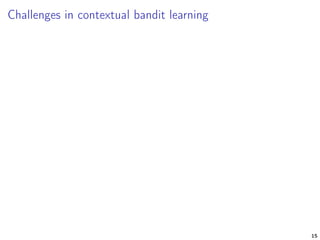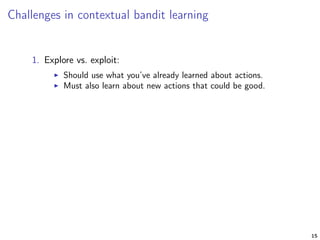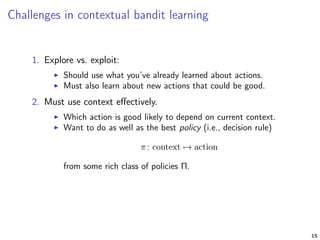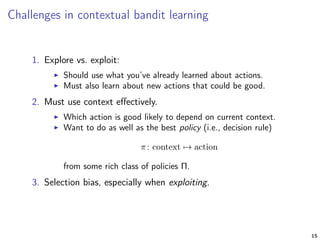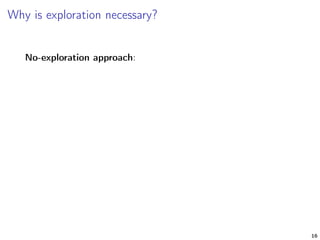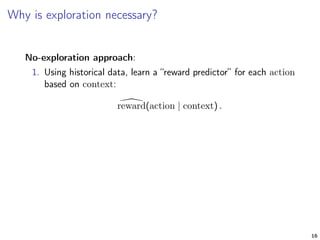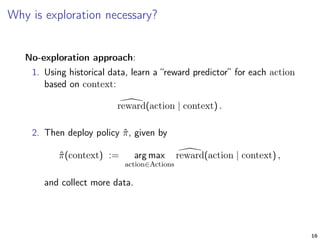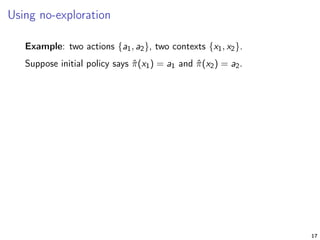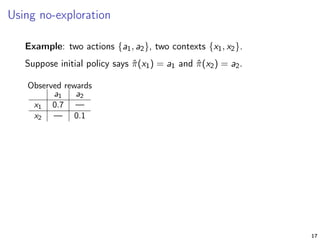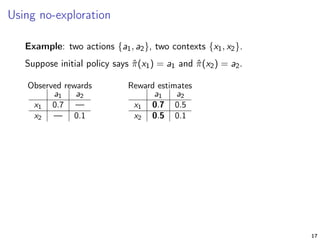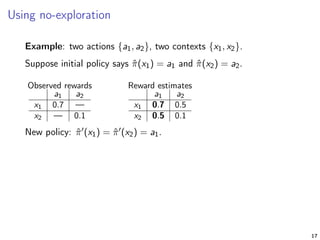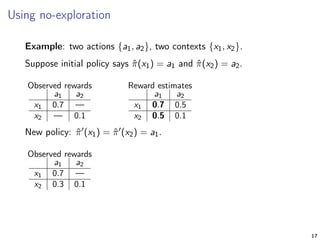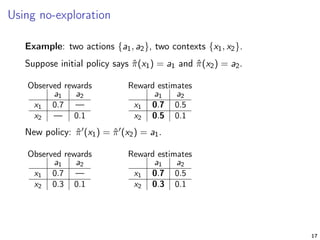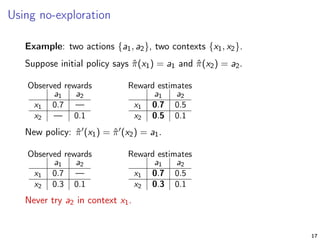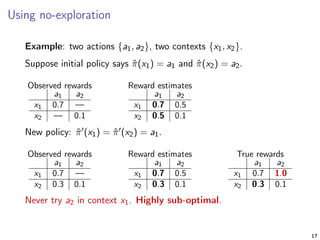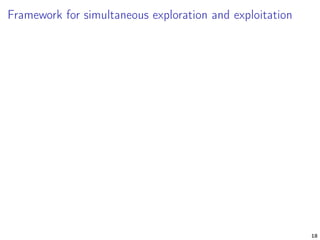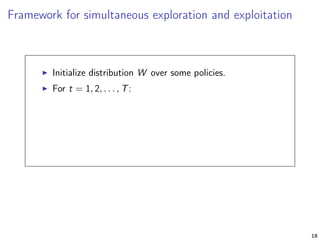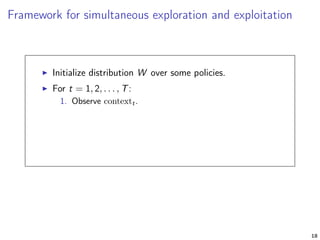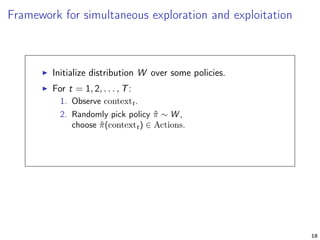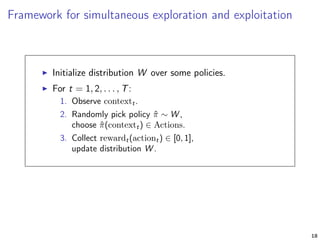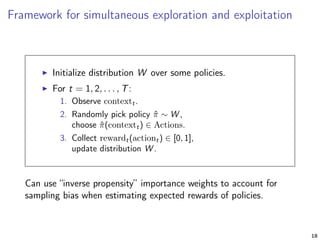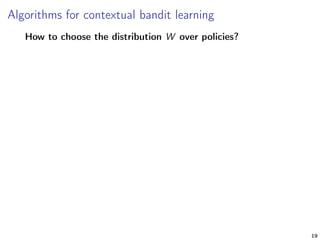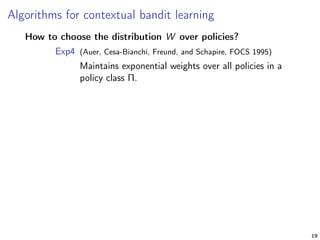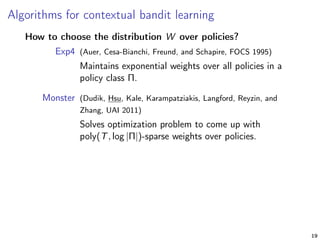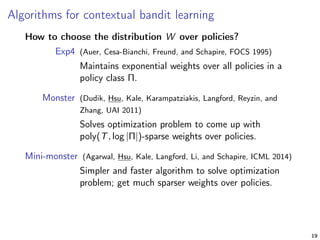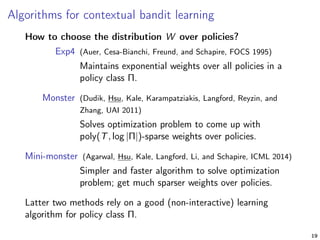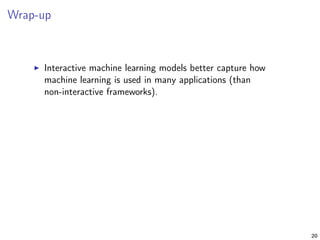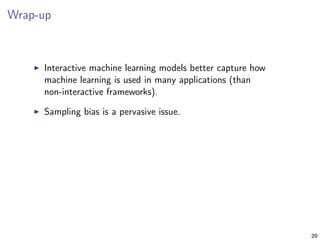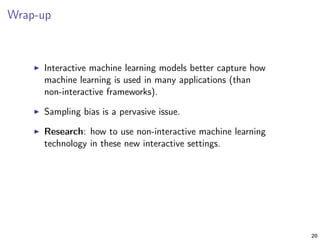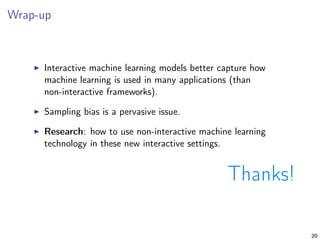1 of 99

### NYAI - Interactive Machine Learning by Daniel Hsu

1. Interactive machine learning Daniel Hsu Columbia University 1
2. Non-interactive machine learning “Cartoon” of (non-interactive) machine learning 2
3. Non-interactive machine learning “Cartoon” of (non-interactive) machine learning 1. Get labeled data {(inputi , outputi )}n i=1. 2
4. Non-interactive machine learning “Cartoon” of (non-interactive) machine learning 1. Get labeled data {(inputi , outputi )}n i=1. 2. Learn prediction function ˆf (e.g., classiﬁer, regressor, policy) such that ˆf (inputi ) ≈ outputi for most i = 1, 2, . . . , n. 2
5. Non-interactive machine learning “Cartoon” of (non-interactive) machine learning 1. Get labeled data {(inputi , outputi )}n i=1. 2. Learn prediction function ˆf (e.g., classiﬁer, regressor, policy) such that ˆf (inputi ) ≈ outputi for most i = 1, 2, . . . , n. Goal: ˆf (input) ≈ output for future (input, output) pairs. 2
6. Non-interactive machine learning “Cartoon” of (non-interactive) machine learning 1. Get labeled data {(inputi , outputi )}n i=1. 2. Learn prediction function ˆf (e.g., classiﬁer, regressor, policy) such that ˆf (inputi ) ≈ outputi for most i = 1, 2, . . . , n. Goal: ˆf (input) ≈ output for future (input, output) pairs. Some applications: document classiﬁcation, face detection, speech recognition, machine translation, credit rating, . . . 2
7. Non-interactive machine learning “Cartoon” of (non-interactive) machine learning 1. Get labeled data {(inputi , outputi )}n i=1. 2. Learn prediction function ˆf (e.g., classiﬁer, regressor, policy) such that ˆf (inputi ) ≈ outputi for most i = 1, 2, . . . , n. Goal: ˆf (input) ≈ output for future (input, output) pairs. Some applications: document classiﬁcation, face detection, speech recognition, machine translation, credit rating, . . . There is a lot of technology for doing this, and a lot of mathematical theories for understanding this. 2
8. Interactive machine learning: example #1 Practicing physician 3
9. Interactive machine learning: example #1 Practicing physician Loop: 1. Patient arrives with symptoms, medical history, genome . . . 3
10. Interactive machine learning: example #1 Practicing physician Loop: 1. Patient arrives with symptoms, medical history, genome . . . 2. Prescribe treatment. 3
11. Interactive machine learning: example #1 Practicing physician Loop: 1. Patient arrives with symptoms, medical history, genome . . . 2. Prescribe treatment. 3. Observe impact on patient’s health (e.g., improves, worsens). 3
12. Interactive machine learning: example #1 Practicing physician Loop: 1. Patient arrives with symptoms, medical history, genome . . . 2. Prescribe treatment. 3. Observe impact on patient’s health (e.g., improves, worsens). Goal prescribe treatments that yield good health outcomes. 3
13. Interactive machine learning: example #2 Website operator 4
14. Interactive machine learning: example #2 Website operator Loop: 1. User visits website with proﬁle, browsing history . . . 4
15. Interactive machine learning: example #2 Website operator Loop: 1. User visits website with proﬁle, browsing history . . . 2. Choose content to display on website. 4
16. Interactive machine learning: example #2 Website operator Loop: 1. User visits website with proﬁle, browsing history . . . 2. Choose content to display on website. 3. Observe user reaction to content (e.g., click, “like”). 4
17. Interactive machine learning: example #2 Website operator Loop: 1. User visits website with proﬁle, browsing history . . . 2. Choose content to display on website. 3. Observe user reaction to content (e.g., click, “like”). Goal choose content that yield desired user behavior. 4
18. Interactive machine learning: example #3 E-mail service provider 5
19. Interactive machine learning: example #3 E-mail service provider Loop: 1. Receive e-mail messages for users (spam or not). 5
20. Interactive machine learning: example #3 E-mail service provider Loop: 1. Receive e-mail messages for users (spam or not). 2. Ask users to provide labels for some borderline cases. 5
21. Interactive machine learning: example #3 E-mail service provider Loop: 1. Receive e-mail messages for users (spam or not). 2. Ask users to provide labels for some borderline cases. 3. Improve spam ﬁlter using newly labeled messages. 5
22. Interactive machine learning: example #3 E-mail service provider Loop: 1. Receive e-mail messages for users (spam or not). 2. Ask users to provide labels for some borderline cases. 3. Improve spam ﬁlter using newly labeled messages. Goal maximize accuracy of spam ﬁlter, minimize number of queries to users. 5
23. Characteristics of interactive machine learning problems 6
24. Characteristics of interactive machine learning problems 1. Learning agent (a.k.a. “learner”) interacts with the world (e.g., patients, users) to gather data. 6
25. Characteristics of interactive machine learning problems 1. Learning agent (a.k.a. “learner”) interacts with the world (e.g., patients, users) to gather data. 2. Learner’s performance based on learner’s decisions. 6
26. Characteristics of interactive machine learning problems 1. Learning agent (a.k.a. “learner”) interacts with the world (e.g., patients, users) to gather data. 2. Learner’s performance based on learner’s decisions. 3. Data available to learner depends on learner’s decisions. 6
27. Characteristics of interactive machine learning problems 1. Learning agent (a.k.a. “learner”) interacts with the world (e.g., patients, users) to gather data. 2. Learner’s performance based on learner’s decisions. 3. Data available to learner depends on learner’s decisions. 4. State of the world depends on learner’s decisions. 6
28. Characteristics of interactive machine learning problems 1. Learning agent (a.k.a. “learner”) interacts with the world (e.g., patients, users) to gather data. 2. Learner’s performance based on learner’s decisions. 3. Data available to learner depends on learner’s decisions. 4. State of the world depends on learner’s decisions. This talk: two interactive machine learning problems, 1. active learning, and 2. contextual bandit learning. (Our models for these problems have all but the last of above characteristics.) 6
29. Active learning
30. Motivation Recall non-interactive (a.k.a. passive) machine learning: 1. Get labeled data {(inputi , labeli )}n i=1. 2. Learn function ˆf (e.g., classiﬁer, regressor, decision rule, policy) such that ˆf (inputi ) ≈ labeli for most i = 1, 2, . . . , n. 8
31. Motivation Recall non-interactive (a.k.a. passive) machine learning: 1. Get labeled data {(inputi , labeli )}n i=1. 2. Learn function ˆf (e.g., classiﬁer, regressor, decision rule, policy) such that ˆf (inputi ) ≈ labeli for most i = 1, 2, . . . , n. Problem: labels often much more expensive to get than inputs. E.g., can’t ask for spam/not-spam label of every e-mail. 8
32. Active learning Basic structure of active learning: 9
33. Active learning Basic structure of active learning: Start with pool of unlabeled inputs (and maybe some (input, label) pairs). 9
34. Active learning Basic structure of active learning: Start with pool of unlabeled inputs (and maybe some (input, label) pairs). Repeat: 1. Train prediction function ˆf using current labeled pairs. 9
35. Active learning Basic structure of active learning: Start with pool of unlabeled inputs (and maybe some (input, label) pairs). Repeat: 1. Train prediction function ˆf using current labeled pairs. 2. Pick some inputs from the pool, and query their labels. 9
36. Active learning Basic structure of active learning: Start with pool of unlabeled inputs (and maybe some (input, label) pairs). Repeat: 1. Train prediction function ˆf using current labeled pairs. 2. Pick some inputs from the pool, and query their labels. Goal: 9
37. Active learning Basic structure of active learning: Start with pool of unlabeled inputs (and maybe some (input, label) pairs). Repeat: 1. Train prediction function ˆf using current labeled pairs. 2. Pick some inputs from the pool, and query their labels. Goal: Final prediction function ˆf satisﬁes ˆf (input) ≈ label for most future (input, label) pairs. 9
38. Active learning Basic structure of active learning: Start with pool of unlabeled inputs (and maybe some (input, label) pairs). Repeat: 1. Train prediction function ˆf using current labeled pairs. 2. Pick some inputs from the pool, and query their labels. Goal: Final prediction function ˆf satisﬁes ˆf (input) ≈ label for most future (input, label) pairs. Number of label queries is small. (Can be selective/adaptive about which labels to query . . . ) 9
39. Sampling bias Main diﬃculty of active learning: sampling bias. 10
40. Sampling bias Main diﬃculty of active learning: sampling bias. At any point in active learning process, labeled data set typically not representative of overall data. 10
41. Sampling bias Main diﬃculty of active learning: sampling bias. At any point in active learning process, labeled data set typically not representative of overall data. Problem can derail learning and go unnoticed! 10
42. Sampling bias Main diﬃculty of active learning: sampling bias. At any point in active learning process, labeled data set typically not representative of overall data. Problem can derail learning and go unnoticed! input ∈ R, label ∈ {0, 1}, classiﬁer type = threshold functions. 45%45% 5%5% 10
43. Sampling bias Typical active learning heuristic: Start with pool of unlabeled inputs. input ∈ R, label ∈ {0, 1}, classiﬁer type = threshold functions. 45%45% 5%5% 10
44. Sampling bias Typical active learning heuristic: Start with pool of unlabeled inputs. Pick a handful of inputs, and query their labels. input ∈ R, label ∈ {0, 1}, classiﬁer type = threshold functions. 45%45% 5%5% 10
45. Sampling bias Typical active learning heuristic: Start with pool of unlabeled inputs. Pick a handful of inputs, and query their labels. Repeat: 1. Train classiﬁer ˆf using current labeled pairs. input ∈ R, label ∈ {0, 1}, classiﬁer type = threshold functions. 45%45% 5%5% 10
46. Sampling bias Typical active learning heuristic: Start with pool of unlabeled inputs. Pick a handful of inputs, and query their labels. Repeat: 1. Train classiﬁer ˆf using current labeled pairs. 2. Pick input from pool closest to decision boundary of ˆf , and query its label. input ∈ R, label ∈ {0, 1}, classiﬁer type = threshold functions. 45%45% 5%5% 10
47. Sampling bias Typical active learning heuristic: Start with pool of unlabeled inputs. Pick a handful of inputs, and query their labels. Repeat: 1. Train classiﬁer ˆf using current labeled pairs. 2. Pick input from pool closest to decision boundary of ˆf , and query its label. input ∈ R, label ∈ {0, 1}, classiﬁer type = threshold functions. 45%45% 5%5% Learner converges to classiﬁer with 5% error rate, even though there’s a classiﬁer with 2.5% error rate. Not statistically consistent! 10
48. Framework for statistically consistent active learning Importance weighted active learning (Beygelzimer, Dasgupta, and Langford, ICML 2009) 11
49. Framework for statistically consistent active learning Importance weighted active learning (Beygelzimer, Dasgupta, and Langford, ICML 2009) Start with unlabeled pool {inputi }n i=1. 11
50. Framework for statistically consistent active learning Importance weighted active learning (Beygelzimer, Dasgupta, and Langford, ICML 2009) Start with unlabeled pool {inputi }n i=1. For each i = 1, 2, . . . , n (in random order): 1. Get inputi . 11
51. Framework for statistically consistent active learning Importance weighted active learning (Beygelzimer, Dasgupta, and Langford, ICML 2009) Start with unlabeled pool {inputi }n i=1. For each i = 1, 2, . . . , n (in random order): 1. Get inputi . 2. Pick probability pi ∈ [0, 1], toss coin with P(heads) = pi . 11
52. Framework for statistically consistent active learning Importance weighted active learning (Beygelzimer, Dasgupta, and Langford, ICML 2009) Start with unlabeled pool {inputi }n i=1. For each i = 1, 2, . . . , n (in random order): 1. Get inputi . 2. Pick probability pi ∈ [0, 1], toss coin with P(heads) = pi . 3. If heads, query labeli , and include (inputi , labeli ) with importance weight 1/pi in data set. 11
53. Framework for statistically consistent active learning Importance weighted active learning (Beygelzimer, Dasgupta, and Langford, ICML 2009) Start with unlabeled pool {inputi }n i=1. For each i = 1, 2, . . . , n (in random order): 1. Get inputi . 2. Pick probability pi ∈ [0, 1], toss coin with P(heads) = pi . 3. If heads, query labeli , and include (inputi , labeli ) with importance weight 1/pi in data set. Importance weight = “inverse propensity” that labeli is queried. Used to account for sampling bias in error rate estimates. 11
54. Importance weighted active learning algorithms How to choose pi ? 12
55. Importance weighted active learning algorithms How to choose pi ? 1. Use your favorite heuristic (as long as pi is never too small). 12
56. Importance weighted active learning algorithms How to choose pi ? 1. Use your favorite heuristic (as long as pi is never too small). 2. Simple rule based on estimated error rate diﬀerences (Beygelzimer, Hsu, Langford, and Zhang, NIPS 2010). 12
57. Importance weighted active learning algorithms How to choose pi ? 1. Use your favorite heuristic (as long as pi is never too small). 2. Simple rule based on estimated error rate diﬀerences (Beygelzimer, Hsu, Langford, and Zhang, NIPS 2010). In many cases, can prove the following: Final classiﬁer is as accurate as learner that queries all n labels. Expected number of queries n i=1 pi grows sub-linearly with n. 12
58. Importance weighted active learning algorithms How to choose pi ? 1. Use your favorite heuristic (as long as pi is never too small). 2. Simple rule based on estimated error rate diﬀerences (Beygelzimer, Hsu, Langford, and Zhang, NIPS 2010). In many cases, can prove the following: Final classiﬁer is as accurate as learner that queries all n labels. Expected number of queries n i=1 pi grows sub-linearly with n. 3. Solve a large convex optimization problem (Huang, Agarwal, Hsu, Langford, and Schapire, NIPS 2015). 12
59. Importance weighted active learning algorithms How to choose pi ? 1. Use your favorite heuristic (as long as pi is never too small). 2. Simple rule based on estimated error rate diﬀerences (Beygelzimer, Hsu, Langford, and Zhang, NIPS 2010). In many cases, can prove the following: Final classiﬁer is as accurate as learner that queries all n labels. Expected number of queries n i=1 pi grows sub-linearly with n. 3. Solve a large convex optimization problem (Huang, Agarwal, Hsu, Langford, and Schapire, NIPS 2015). Even stronger theoretical guarantees. 12
60. Importance weighted active learning algorithms How to choose pi ? 1. Use your favorite heuristic (as long as pi is never too small). 2. Simple rule based on estimated error rate diﬀerences (Beygelzimer, Hsu, Langford, and Zhang, NIPS 2010). In many cases, can prove the following: Final classiﬁer is as accurate as learner that queries all n labels. Expected number of queries n i=1 pi grows sub-linearly with n. 3. Solve a large convex optimization problem (Huang, Agarwal, Hsu, Langford, and Schapire, NIPS 2015). Even stronger theoretical guarantees. Latter two methods can be made to work with any classiﬁer type, provided a good (non-interactive) learning algorithm. 12
61. Contextual bandit learning
62. Contextual bandit learning 14
63. Contextual bandit learning Protocol for contextual bandit learning: For round t = 1, 2, . . . , T: 14
64. Contextual bandit learning Protocol for contextual bandit learning: For round t = 1, 2, . . . , T: 1. Observe contextt. [e.g., user proﬁle] 14
65. Contextual bandit learning Protocol for contextual bandit learning: For round t = 1, 2, . . . , T: 1. Observe contextt. [e.g., user proﬁle] 2. Choose actiont ∈ Actions. [e.g., display ad] 14
66. Contextual bandit learning Protocol for contextual bandit learning: For round t = 1, 2, . . . , T: 1. Observe contextt. [e.g., user proﬁle] 2. Choose actiont ∈ Actions. [e.g., display ad] 3. Collect rewardt(actiont) ∈ [0, 1]. [e.g., click or no-click] 14
67. Contextual bandit learning Protocol for contextual bandit learning: For round t = 1, 2, . . . , T: 1. Observe contextt. [e.g., user proﬁle] 2. Choose actiont ∈ Actions. [e.g., display ad] 3. Collect rewardt(actiont) ∈ [0, 1]. [e.g., click or no-click] Goal: Choose actions that yield high reward. 14
68. Contextual bandit learning Protocol for contextual bandit learning: For round t = 1, 2, . . . , T: 1. Observe contextt. [e.g., user proﬁle] 2. Choose actiont ∈ Actions. [e.g., display ad] 3. Collect rewardt(actiont) ∈ [0, 1]. [e.g., click or no-click] Goal: Choose actions that yield high reward. Note: In round t, only observe reward for chosen actiont, and not reward that you would’ve received if you’d chosen a diﬀerent action. 14
69. Challenges in contextual bandit learning 15
70. Challenges in contextual bandit learning 1. Explore vs. exploit: Should use what you’ve already learned about actions. Must also learn about new actions that could be good. 15
71. Challenges in contextual bandit learning 1. Explore vs. exploit: Should use what you’ve already learned about actions. Must also learn about new actions that could be good. 2. Must use context eﬀectively. Which action is good likely to depend on current context. Want to do as well as the best policy (i.e., decision rule) π: context → action from some rich class of policies Π. 15
72. Challenges in contextual bandit learning 1. Explore vs. exploit: Should use what you’ve already learned about actions. Must also learn about new actions that could be good. 2. Must use context eﬀectively. Which action is good likely to depend on current context. Want to do as well as the best policy (i.e., decision rule) π: context → action from some rich class of policies Π. 3. Selection bias, especially when exploiting. 15
73. Why is exploration necessary? No-exploration approach: 16
74. Why is exploration necessary? No-exploration approach: 1. Using historical data, learn a “reward predictor” for each action based on context: reward(action | context) . 16
75. Why is exploration necessary? No-exploration approach: 1. Using historical data, learn a “reward predictor” for each action based on context: reward(action | context) . 2. Then deploy policy ˆπ, given by ˆπ(context) := arg max action∈Actions reward(action | context) , and collect more data. 16
76. Using no-exploration Example: two actions {a1, a2}, two contexts {x1, x2}. Suppose initial policy says ˆπ(x1) = a1 and ˆπ(x2) = a2. 17
77. Using no-exploration Example: two actions {a1, a2}, two contexts {x1, x2}. Suppose initial policy says ˆπ(x1) = a1 and ˆπ(x2) = a2. Observed rewards a1 a2 x1 0.7 — x2 — 0.1 17
78. Using no-exploration Example: two actions {a1, a2}, two contexts {x1, x2}. Suppose initial policy says ˆπ(x1) = a1 and ˆπ(x2) = a2. Observed rewards a1 a2 x1 0.7 — x2 — 0.1 Reward estimates a1 a2 x1 0.7 0.5 x2 0.5 0.1 17
79. Using no-exploration Example: two actions {a1, a2}, two contexts {x1, x2}. Suppose initial policy says ˆπ(x1) = a1 and ˆπ(x2) = a2. Observed rewards a1 a2 x1 0.7 — x2 — 0.1 Reward estimates a1 a2 x1 0.7 0.5 x2 0.5 0.1 New policy: ˆπ (x1) = ˆπ (x2) = a1. 17
80. Using no-exploration Example: two actions {a1, a2}, two contexts {x1, x2}. Suppose initial policy says ˆπ(x1) = a1 and ˆπ(x2) = a2. Observed rewards a1 a2 x1 0.7 — x2 — 0.1 Reward estimates a1 a2 x1 0.7 0.5 x2 0.5 0.1 New policy: ˆπ (x1) = ˆπ (x2) = a1. Observed rewards a1 a2 x1 0.7 — x2 0.3 0.1 17
81. Using no-exploration Example: two actions {a1, a2}, two contexts {x1, x2}. Suppose initial policy says ˆπ(x1) = a1 and ˆπ(x2) = a2. Observed rewards a1 a2 x1 0.7 — x2 — 0.1 Reward estimates a1 a2 x1 0.7 0.5 x2 0.5 0.1 New policy: ˆπ (x1) = ˆπ (x2) = a1. Observed rewards a1 a2 x1 0.7 — x2 0.3 0.1 Reward estimates a1 a2 x1 0.7 0.5 x2 0.3 0.1 17
82. Using no-exploration Example: two actions {a1, a2}, two contexts {x1, x2}. Suppose initial policy says ˆπ(x1) = a1 and ˆπ(x2) = a2. Observed rewards a1 a2 x1 0.7 — x2 — 0.1 Reward estimates a1 a2 x1 0.7 0.5 x2 0.5 0.1 New policy: ˆπ (x1) = ˆπ (x2) = a1. Observed rewards a1 a2 x1 0.7 — x2 0.3 0.1 Reward estimates a1 a2 x1 0.7 0.5 x2 0.3 0.1 Never try a2 in context x1. 17
83. Using no-exploration Example: two actions {a1, a2}, two contexts {x1, x2}. Suppose initial policy says ˆπ(x1) = a1 and ˆπ(x2) = a2. Observed rewards a1 a2 x1 0.7 — x2 — 0.1 Reward estimates a1 a2 x1 0.7 0.5 x2 0.5 0.1 New policy: ˆπ (x1) = ˆπ (x2) = a1. Observed rewards a1 a2 x1 0.7 — x2 0.3 0.1 Reward estimates a1 a2 x1 0.7 0.5 x2 0.3 0.1 True rewards a1 a2 x1 0.7 1.0 x2 0.3 0.1 Never try a2 in context x1. Highly sub-optimal. 17
84. Framework for simultaneous exploration and exploitation 18
85. Framework for simultaneous exploration and exploitation Initialize distribution W over some policies. For t = 1, 2, . . . , T: 18
86. Framework for simultaneous exploration and exploitation Initialize distribution W over some policies. For t = 1, 2, . . . , T: 1. Observe contextt. 18
87. Framework for simultaneous exploration and exploitation Initialize distribution W over some policies. For t = 1, 2, . . . , T: 1. Observe contextt. 2. Randomly pick policy ˆπ ∼ W , choose ˆπ(contextt) ∈ Actions. 18
88. Framework for simultaneous exploration and exploitation Initialize distribution W over some policies. For t = 1, 2, . . . , T: 1. Observe contextt. 2. Randomly pick policy ˆπ ∼ W , choose ˆπ(contextt) ∈ Actions. 3. Collect rewardt(actiont) ∈ [0, 1], update distribution W . 18
89. Framework for simultaneous exploration and exploitation Initialize distribution W over some policies. For t = 1, 2, . . . , T: 1. Observe contextt. 2. Randomly pick policy ˆπ ∼ W , choose ˆπ(contextt) ∈ Actions. 3. Collect rewardt(actiont) ∈ [0, 1], update distribution W . Can use “inverse propensity” importance weights to account for sampling bias when estimating expected rewards of policies. 18
90. Algorithms for contextual bandit learning How to choose the distribution W over policies? 19
91. Algorithms for contextual bandit learning How to choose the distribution W over policies? Exp4 (Auer, Cesa-Bianchi, Freund, and Schapire, FOCS 1995) Maintains exponential weights over all policies in a policy class Π. 19
92. Algorithms for contextual bandit learning How to choose the distribution W over policies? Exp4 (Auer, Cesa-Bianchi, Freund, and Schapire, FOCS 1995) Maintains exponential weights over all policies in a policy class Π. Monster (Dudik, Hsu, Kale, Karampatziakis, Langford, Reyzin, and Zhang, UAI 2011) Solves optimization problem to come up with poly(T, log |Π|)-sparse weights over policies. 19
93. Algorithms for contextual bandit learning How to choose the distribution W over policies? Exp4 (Auer, Cesa-Bianchi, Freund, and Schapire, FOCS 1995) Maintains exponential weights over all policies in a policy class Π. Monster (Dudik, Hsu, Kale, Karampatziakis, Langford, Reyzin, and Zhang, UAI 2011) Solves optimization problem to come up with poly(T, log |Π|)-sparse weights over policies. Mini-monster (Agarwal, Hsu, Kale, Langford, Li, and Schapire, ICML 2014) Simpler and faster algorithm to solve optimization problem; get much sparser weights over policies. 19
94. Algorithms for contextual bandit learning How to choose the distribution W over policies? Exp4 (Auer, Cesa-Bianchi, Freund, and Schapire, FOCS 1995) Maintains exponential weights over all policies in a policy class Π. Monster (Dudik, Hsu, Kale, Karampatziakis, Langford, Reyzin, and Zhang, UAI 2011) Solves optimization problem to come up with poly(T, log |Π|)-sparse weights over policies. Mini-monster (Agarwal, Hsu, Kale, Langford, Li, and Schapire, ICML 2014) Simpler and faster algorithm to solve optimization problem; get much sparser weights over policies. Latter two methods rely on a good (non-interactive) learning algorithm for policy class Π. 19
95. Wrap-up 20
96. Wrap-up Interactive machine learning models better capture how machine learning is used in many applications (than non-interactive frameworks). 20
97. Wrap-up Interactive machine learning models better capture how machine learning is used in many applications (than non-interactive frameworks). Sampling bias is a pervasive issue. 20
98. Wrap-up Interactive machine learning models better capture how machine learning is used in many applications (than non-interactive frameworks). Sampling bias is a pervasive issue. Research: how to use non-interactive machine learning technology in these new interactive settings. 20
99. Wrap-up Interactive machine learning models better capture how machine learning is used in many applications (than non-interactive frameworks). Sampling bias is a pervasive issue. Research: how to use non-interactive machine learning technology in these new interactive settings. Thanks! 20